﻿ Digital Circuits and Systems - Circuits i Sistemes Digitals (CSD) - EETAC - UPCBachelor's Degree in Telecommunications Systems and in Network Engineering## P4: Arithmetic operations for signed integers in 2C. Measuring propagation delay and calculation speed

 Resources in lectures and labs: L4.1, Lab4, L4.2,  L4.3 Project objectives

Highlighted project: 8-bit adder-subtractor for signed integers. How fast is this circuit?

1. Specifications

Design an 8-bit adder and subtractor in two's complement with zero (Z), sign (S) and overflow (OV) flags using VHDL and multiple-file hierarchical structural approach (plan C2). Discus the symbol (below in Fig 1). Write its truth table. How long is it? Draw a timing diagram example.Fig. 1. a) Symbol of an 8-bit two's complement(2C) adder/subtractor.     Run this project solved in Proteus Int_add_subt_8bit.pdsprj and try to visualise better how operates and how the two's complemented data looks like.b) Example of truth table combinations. Int_add_subt_8bit works only with signed integer operands.c) Timing diagram sketch showing how the Int_Add_Subt_8bit performs several additions and subtractions using two's complement signed integer operands. It is ready for translation as a VHDL testbench stimulus process.

Circuit's maximum processing speed. Once the circuit works and is tested functionally as usual, perform a gate-level simulation to determine the circuit's propagation delay in a given transition and also the circuit's maximum speed of operation using the timer analyser tool.

VHDL design flow shows gate-level simulation as the 5th design step before making real measurements in the lab uploading the configuration file to the prototyping board. For instance, two different experiments can be carried out:

 Measure computation speed of different circuits synthesised in the same target chip, for instance, MAX II EPM2210F324C3. Logically, longer circuits composed of more levels of gates generate larger propagation delays and thus calculate slower.  Adder_1bit Adder_Subt_8bit You can also discuss how the electrical characteristics of the synthesised circuit are dependent on technology changing the target chip. Measure maximum speed of operation of Adder_Subt_8bit using (each circuit is a different project): Board Terasic -DE2-115 populated with Cyclone IV EP4CE115F29C7 Board Terasic MAX II Micro Kit using EPM2210F324C3

Other hierarchical multiple-file arithmetic 2C projects:

- Selectable_mult_8bit in Proteus, 8-bit multiplier for  integer in two's complement and radix-2 numbers.

- Int_Comp_8bit, 8-bit comparator for integer numbers in two's complement.

- ALU_4bit

Other assignments (design problems D1.1, D1.2, ...)

2. Planning circuits using hierarchical multiple-component structure

We can deduce the plan for this circuit studying the algorithm behind results and flags. The idea is to reuse for both, additions and subtractions a single Adder_8bit for radix-2 numbers found in P3.Fig. 2. XOR gate examined as a configurable NOT or buffer.

- Study how the zero flag (Z) works. Is it the same as in Adder_8bit? (Int_add_subt_8bit Z flag does not include Cout signal)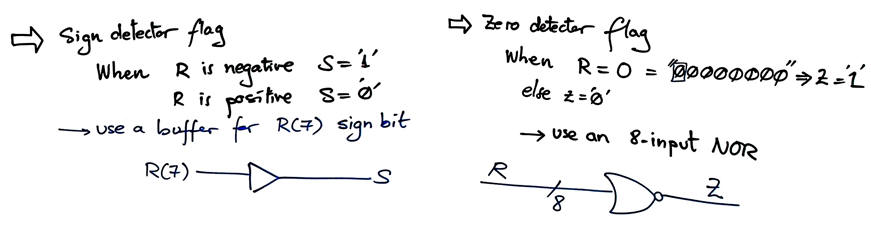Fig. 3. Circuits for sign (S) and zero (Z) flags. If Adder_4bit from P3 is to be used, we have to modify it, so that Z equation is not including Cout, thus: Z = (Y3 + Y2 + Y1 + Y0)'

Study how the overflow logic (OV) works and how a circuit can be inferred.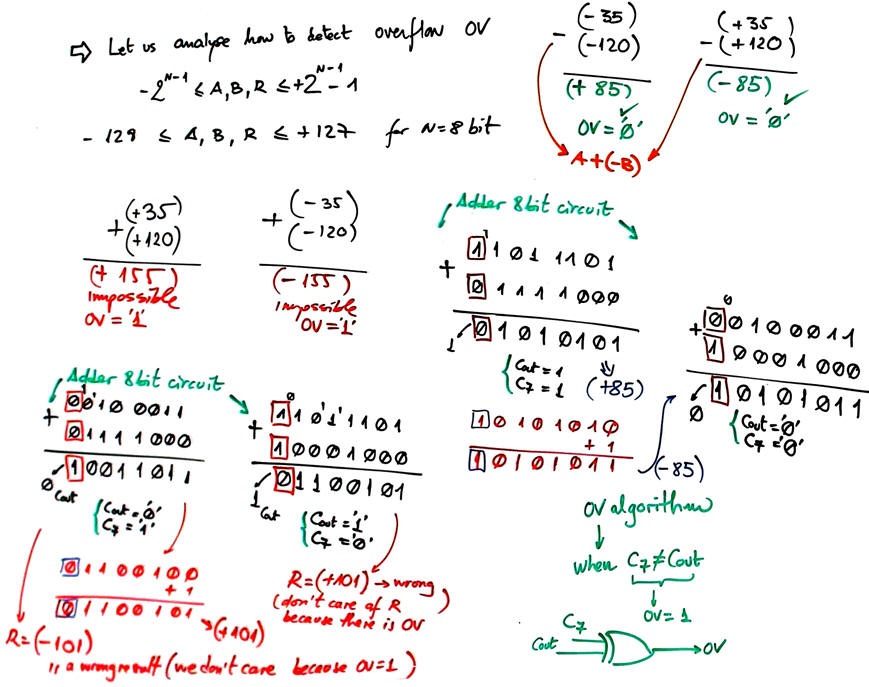Fig. 4. On the detection of the overflow condition. Carry C7 has to be made available from the Adder_8bit.

Final plan C2 for Int_Add_Subt_8bit is represented in Fig. 5.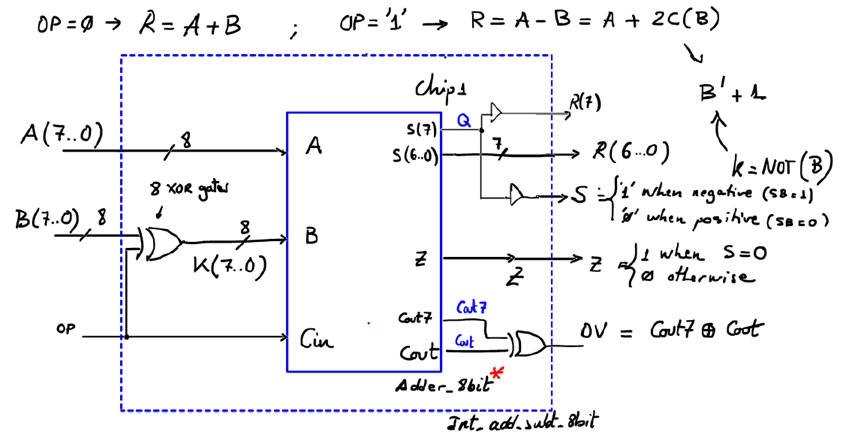Fig. 5. Proposed plan.

Count and name all the components and VHDL files involved in the project.

Project folder location:

3. Development

Once you have completed the planning of the project in Fig. 5, you can start the synthesis process. Write down the VHDL files translating the plans above using components and signals modifying a convenient seed circuit. For instance, these circuits may suit you: Adder_1bit.vhd, Adder_4bit.vhd, Adder_8bit.vhd, Int_add_subt_8bit.vhd. You see that the 4-bit and 8-bit adders are slightly modified because the overflow flag OV is generated using the most significant carry signals.

Run the EDA tool to synthesise the circuit. Print and comment the RTL schematic. Is it like what you had sketched in the plan?Fig. 6. Example of RTL diagram schematic interpreted by the EDA synthesis tool.

At this level, it is very interesting for you to play with technology schematics as well. They are the real synthesised circuits for a given target chip. In addition to the technology view, there is also the chip planner tool where you can locate exactly the logic cells used in the design.Fig. 7. Example of technology implementation in an FPGA Cyclone IV EP4CE115F29C7 from Intel. In this example circuit, 21 logic elements are used of the 114480 available. Remark how no memory registers/latches are used because it is a combinational circuit (Chapter I) instead of a sequential system (Chapter II). In this sense, if your implementation contains registers or inferred latches it means that it is wrong and you must debug your architecture to find mistakes in VHDL files.

4. Testing (functional simulation)

The testbench fixture containing the main ideas and concepts involved in this schematic is represented in Fig. 8.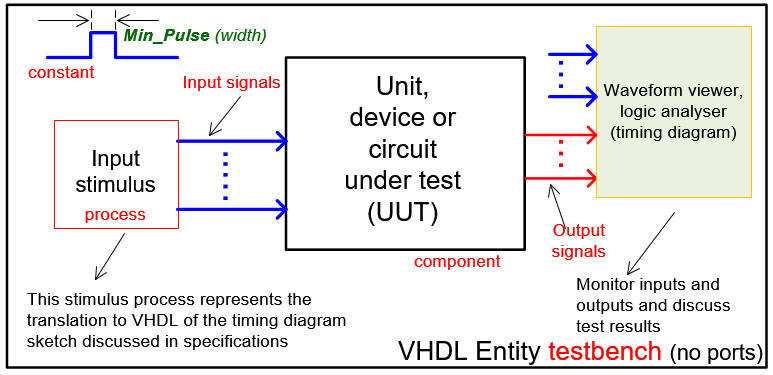Fig. 8. Testbench VHDL schematic.

Generate a testbench template from Quartus Prime. Rename and move it to the project folder. Delete the empty stimulus process.

Convert the initial timing diagram sketch in Fig. 1c into a VHDL testbench. For example, copy only the stimulus process and the constant Min_Pulse from this file: Int_add_subt_8bit_tb.vhd. Use several positive and negative integer numbers as inputs. Test both additions and subtractions. Check overflow and zero situations.

Run the EDA VHDL simulator and demonstrate how the circuit works adding comments to the printed sheet of paper containing the waveforms, as shown in Fig. 9.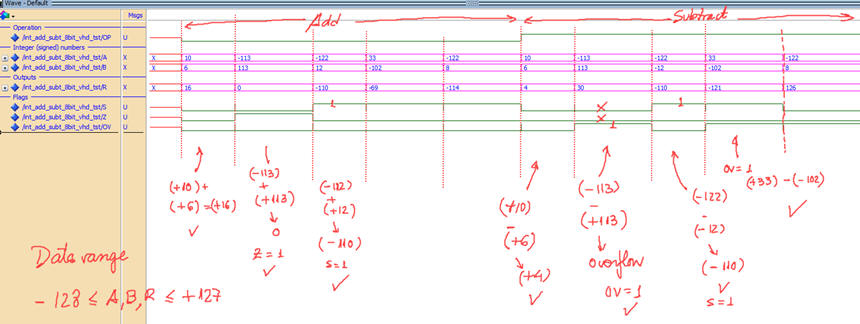Fig. 9. Example test with some input vectors. A, B and R are 2C numbers (radix decimal allows identifying  correctly the binary numbers as signed integers).

5. Testing (gate-level simulation) and timing analyser measurements

Two tools for dynamic characterisation: gate-level ModelSim simulations and Quartus Prime timing analyser.

### Gate-level simulations

Run a to measure the worst case delay and the maximum speed of operation (or highest computation speed) of the synthesised circuit.

Fig. 10 shows the new simulation project, considering the testbench and the flat technology view schematic translated to VHDL by Quartus Prime as the Int_Add_Subt_8bit.vho file.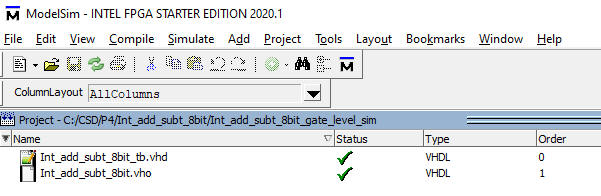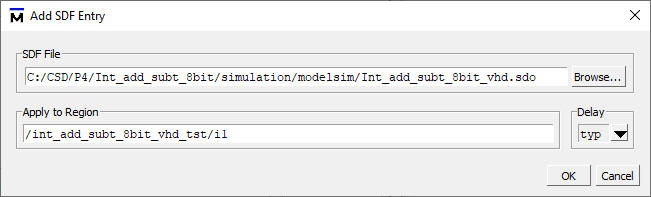Fig. 10. Gate-level project files. Attach the SDF file to the simulation.

Perform gate-level simulations on several input vectors examining (zooming in) signal transitions. Measure the circuit propagation delay.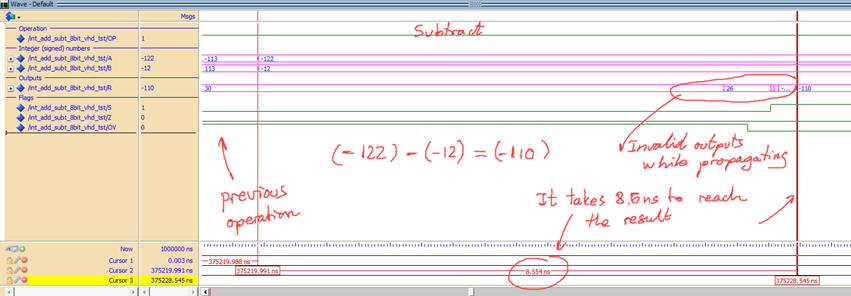Fig. 11. Example waveform for the Int_add_subt_8bitshowing how a given input vector is computed over time generating wrong results until all signals have propagated through the circuit. Target chip: Cyclone IV FPGA EP4CE115F29C7.

When Min_Pulse constant is similar to propagation times the circuit cannot produce correct results.a)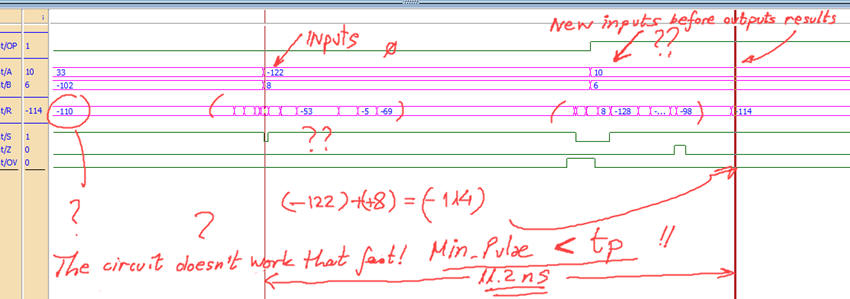b) Fig. 12. (a) Results for Min_Pulse = 20 ns, the circuit still operating correctly. (b) Results for Min_Pulse = 8 ns, too fast, new input stimulus is applied before the circuit sets the outputs of the previous operation.

### Timing analyser

Use the Quartus Prime timing analyser tool to determine the longest propagation delay path from inputs to outputs (worst-case scenario), and thus the maximum speed for calculating 8-bit integer additions and subtractions for a given target chip. For instance, considering Intel chips, which is faster, MAX II CPLD EPM2210F324C3 or Cyclone IV FPGA EP4CE115F29C7N ?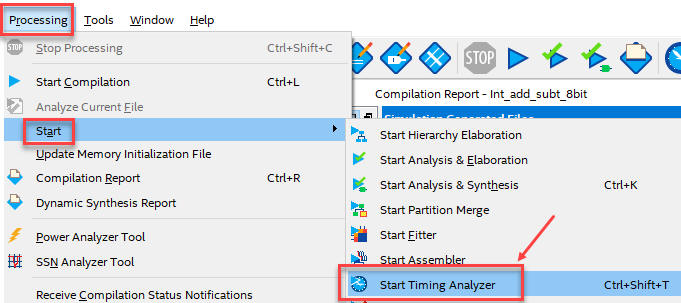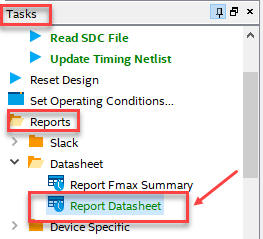Fig. 13. Processing the timing analyser spreadsheet engine and reporting datasheet results.

As shown in Fig. 14, switching OP from H to L (falling edge) and waiting for setting the Z flag from H to L (falling edge), generates the maximum propagation delay tP = 14.691 ns.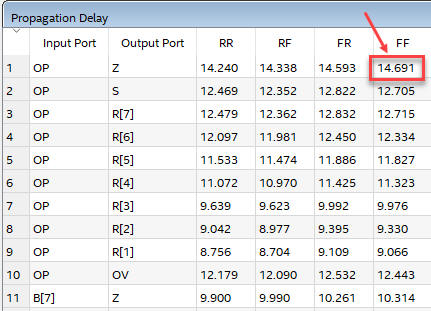Fig. 14. A section of the timing analyser spreadsheet showing the worst-case scenario.

Therefore, if input OP has a switching period T, its minimum value is Tmin = 2·tP = 29.34 ns. In this circuit the maximum speed is fMAX <  34 MHz (34 millions of operations per second).

6. Report

It is required a handwritten original project report containing sections 1 - 2 - 3 - 4 -5, scanned figures with annotations, file listings, diagrams, sketches or any other resources. Theory to comprehend how the circuit works and how two's complement data operates may be included in section 1 on specifications. Follow this for writing reports.

7. Prototyping

Use training boards and perform laboratory measurements to verify how the circuit being designed works for real.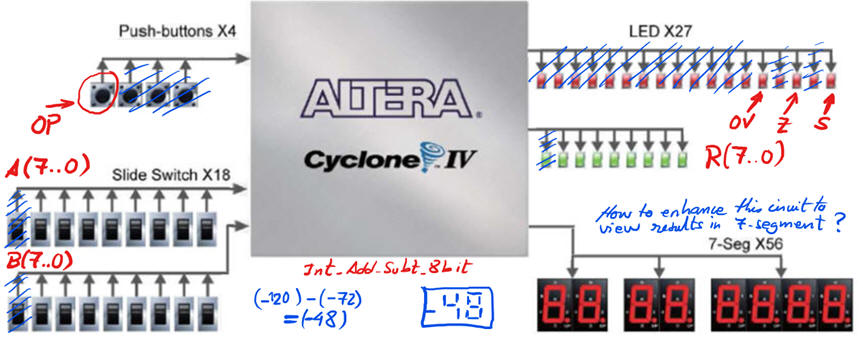Fig. 15. Switches are used for A(7..0) and B(7..0) operands. One button OP selects add or subtract. Eight green LED are used to represent signed integer results R(7..0), and three red LED are used for displaying flags.